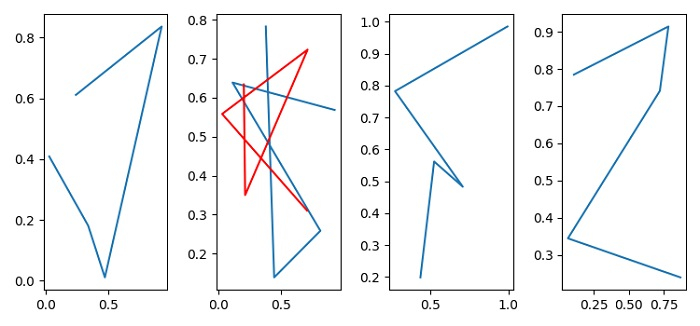# How can I programmatically select a specific subplot in Matplotlib?

To select a specific subplot in matplotlib, we can take the following steps −

• Set the figure size and adjust the padding between and around the subplots.

• Create a new figure or activate an existing figure using figure() method.

• Iterate in a range, i.e., number subplots to be placed.

• In the loop itself, add an '~.axes.Axes' to the figure as part of a subplot arrangement using add_subplot() method.

• Now, select an axes plot line with red color.

• To display the figure, use show() method.

## Example

import numpy as np
import matplotlib.pyplot as plt

plt.rcParams["figure.figsize"] = [7.50, 3.50]
plt.rcParams["figure.autolayout"] = True

fig = plt.figure()

for index in [1, 2, 3, 4]:
plt.show()